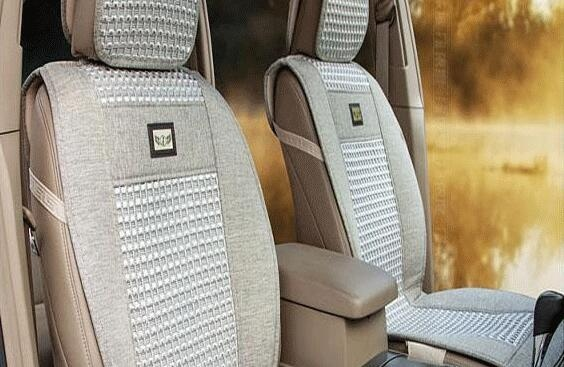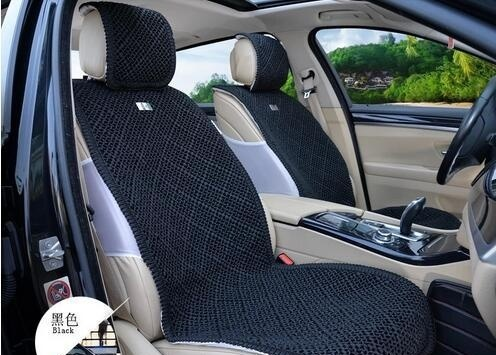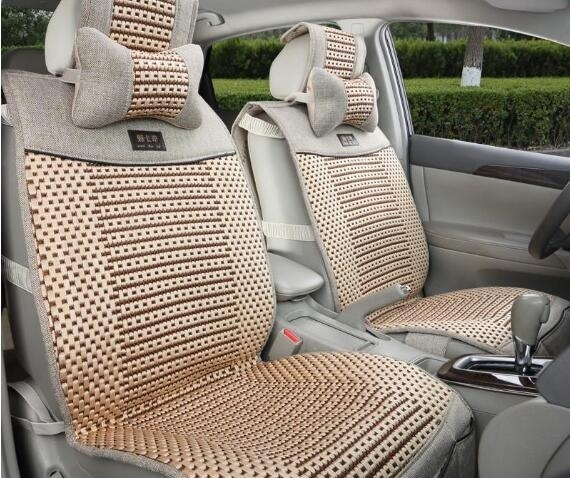|

# 亚麻汽车坐垫哪个牌子好 真假亚麻汽车坐垫鉴别

汽车坐垫品牌多种类多，亚麻汽车坐垫相信大家也听说过的，亚麻汽车坐垫是由天然纤维，亚麻纤维提炼而成，纯天然无污染，强韧、强度大、色泽好、柔细，亚麻汽车坐垫哪个牌子好？真假亚麻汽车坐垫如何鉴别？接下来就为大家具体解析一下。亚麻汽车坐垫哪个牌子好

亚麻汽车坐垫品牌

澳纯的这款汽车坐垫采用的是百分百天然纤维亚麻纤维编织成的，它的这款汽车坐垫采用的是3D立体背部调整坐姿，采用荞麦壳制作成按摩条，有助于缓解疲劳。蝴蝶头枕防止头部偏移，大号的靠腰垫，减少腰部的压力。采用的是防滑底部，能有效的全方位固定坐垫，在驾驶过程中不受到一点影响。采用的是天然荞麦壳为填充物，使得坐垫超强透气性好，又无毒无害，环保有益于身体健康。这款亚麻汽车坐垫适合一般无座通用车。

亚麻汽车坐垫品牌

金澳兰德这款汽车坐垫是采用优质的天然亚麻，汽车坐垫的两侧采用的是双层包边设计，线条流畅，层次分明。它的坐感舒服，两侧双层设计师坐垫左右想中间倾斜，自然包裹后背和臀部，有效的缓解驾驶疲劳感。采用的是名贵中药味，决明子和荞麦壳为填充物。配合冰麻材料，药物能直接作用于人体，起到保健养生的功效。它的这款亚麻汽车坐垫颜色丰富，色彩艳丽，是女士爱车不错的选择。

亚麻汽车坐垫品牌 这款汽车坐垫不适来自艾金诺亚麻汽车坐垫 ，这款采用的是颜色拼接的款式，英伦风格的坐垫。这款汽车坐垫后背人性化的设计，方便收纳闲置的物品，提高空间利用率。防滑口的设计，不仅提升整体的美观，又使坐垫牢固不滑落。这款汽车坐垫采用的是百分百亚麻面料，款式时尚，人性化的设计，是时尚一族不错的选择。真假亚麻汽车坐垫鉴别

一、眼观法：

100％纯手编汽车亚麻坐垫：光泽度均匀，要比同款的亚麻含量较少的麻棉坐垫亮一些。

麻棉坐垫：颜色和亚麻一样只有灰色和亮白色，但相比纯亚麻坐垫相对暗淡，灰色大多偏黑。不如纯亚麻坐垫光泽度均匀。

冰丝光泽度强，颜色光鲜亮丽且没有明显瑕点。

二、手摸法：

纯亚麻产品：弹性小，强度比棉线好，手感比棉线硬，用力握粗糙的摩擦感。夏季手摸亚麻坐垫，感觉温度降低3－5度。

化纤制品摸起来较为光滑。

三、燃烧法：

取一点亚麻织物的纱线，亚麻纤维燃烧时速度缓慢，燃烧后有燃纸味，灰烬细腻呈白灰色，

棉麻纤维燃烧迅速快，燃烧后为黑灰。

冰丝织物燃烧后为黑渣状。化纤燃烧有刺激性气味冒黑烟，且灰烬呈黑色硬颗粒状。

四、比重法：

由于亚麻比其他材质更重更硬，因此亚麻坐垫的含量是和重量成正比的。亚麻含量越高，坐垫越重。

五、比价法：

100％纯亚麻坐垫价格是不会便宜的，一般至少在800元到上千元，目前市场上三四百元的坐垫多为亚麻和其它织物混纺而成，因此购买时不能仅凭价格判断，要综合分析坐垫的亚麻含量和制作工艺，再决定是否购买。

六、看清洗液：

亚麻坐垫：比较不容易清洗，且洗后干燥方法不正确有时会泛黄，因此厂家多以深色调为主，并赠送有亚麻坐垫专用清洗液。

冰丝及混纺坐垫：可以随意清洗，洗后没有什么大的变化。厂家一般不会配备坐垫专用清洗液。亚麻汽车坐垫好吗

亚麻属于一种稀有的纯天然的植物，它的纤维也是人类最早使用的天然物质之一。它拥有很强的韧性，柔而细、色泽美观可以调控、遇水不易融化等特点。亚麻汽车坐垫也是众多司机们的最爱，拥有天然空调的美誉。

亚麻汽车坐垫易清洗保养，清洗时注意些什么？

1、由于材质的不融水性，清洗选择广泛可以手洗、洗衣机清洗、干洗等；

2、保养的方法：由于清洗方式的多样化，用水洗的过程中先要浸泡10分钟左右。清洗过程中不要用力拧干，最好选择铺平放到阴凉地使其自然干。用洗衣机洗时洗完不能脱水，阴干过后才进行高温熨烫。由于柔软的物理性质，晒干过后可以叠放到很小空间里面存放；

3、清洗的过程中要注意了！水的温度不易过高，最好控制在35至40摄氏度。在清洗的过程中选择的洗涤液最好是用中性的，不要用高效漂白类型的。最好不要和酸性的物质接触，不然很容易破坏纤维组织使其烧坏。

`声明：本文由入驻焦点开放平台的作者撰写，除焦点官方账号外，观点仅代表作者本人，不代表焦点立场错误信息举报电话： 400-099-0099，邮箱：jubao@vip.sohu.com，或点此进行意见反馈，或点此进行举报投诉。`A B C D E F G H J K L M N P Q R S T W X Y Z
A - B - C - D - E
• A
• 鞍山
• 安庆
• 安阳
• 安顺
• 安康
• 澳门
• B
• 北京
• 保定
• 包头
• 巴彦淖尔
• 本溪
• 蚌埠
• 亳州
• 滨州
• 北海
• 百色
• 巴中
• 毕节
• 保山
• 宝鸡
• 白银
• 巴州
• C
• 承德
• 沧州
• 长治
• 赤峰
• 朝阳
• 长春
• 常州
• 滁州
• 池州
• 长沙
• 常德
• 郴州
• 潮州
• 崇左
• 重庆
• 成都
• 楚雄
• 昌都
• 慈溪
• 常熟
• D
• 大同
• 大连
• 丹东
• 大庆
• 东营
• 德州
• 东莞
• 德阳
• 达州
• 大理
• 德宏
• 定西
• 儋州
• 东平
• E
• 鄂尔多斯
• 鄂州
• 恩施
F - G - H - I - J
• F
• 抚顺
• 阜新
• 阜阳
• 福州
• 抚州
• 佛山
• 防城港
• G
• 赣州
• 广州
• 桂林
• 贵港
• 广元
• 广安
• 贵阳
• 固原
• H
• 邯郸
• 衡水
• 呼和浩特
• 呼伦贝尔
• 葫芦岛
• 哈尔滨
• 黑河
• 淮安
• 杭州
• 湖州
• 合肥
• 淮南
• 淮北
• 黄山
• 菏泽
• 鹤壁
• 黄石
• 黄冈
• 衡阳
• 怀化
• 惠州
• 河源
• 贺州
• 河池
• 海口
• 红河
• 汉中
• 海东
• I
• J
• 晋中
• 锦州
• 吉林
• 鸡西
• 佳木斯
• 嘉兴
• 金华
• 景德镇
• 九江
• 吉安
• 济南
• 济宁
• 焦作
• 荆门
• 荆州
• 江门
• 揭阳
• 金昌
• 酒泉
• 嘉峪关
K - L - M - N - P
• K
• 开封
• 昆明
• 昆山
• L
• 廊坊
• 临汾
• 辽阳
• 连云港
• 丽水
• 六安
• 龙岩
• 莱芜
• 临沂
• 聊城
• 洛阳
• 漯河
• 娄底
• 柳州
• 来宾
• 泸州
• 乐山
• 六盘水
• 丽江
• 临沧
• 拉萨
• 林芝
• 兰州
• 陇南
• M
• 牡丹江
• 马鞍山
• 茂名
• 梅州
• 绵阳
• 眉山
• N
• 南京
• 南通
• 宁波
• 南平
• 宁德
• 南昌
• 南阳
• 南宁
• 内江
• 南充
• P
• 盘锦
• 莆田
• 平顶山
• 濮阳
• 攀枝花
• 普洱
• 平凉
Q - R - S - T - W
• Q
• 秦皇岛
• 齐齐哈尔
• 衢州
• 泉州
• 青岛
• 清远
• 钦州
• 黔南
• 曲靖
• 庆阳
• R
• 日照
• 日喀则
• S
• 石家庄
• 沈阳
• 双鸭山
• 绥化
• 上海
• 苏州
• 宿迁
• 绍兴
• 宿州
• 三明
• 上饶
• 三门峡
• 商丘
• 十堰
• 随州
• 邵阳
• 韶关
• 深圳
• 汕头
• 汕尾
• 三亚
• 三沙
• 遂宁
• 山南
• 商洛
• 石嘴山
• T
• 天津
• 唐山
• 太原
• 通辽
• 铁岭
• 泰州
• 台州
• 铜陵
• 泰安
• 铜仁
• 铜川
• 天水
• 天门
• W
• 乌海
• 乌兰察布
• 无锡
• 温州
• 芜湖
• 潍坊
• 威海
• 武汉
• 梧州
• 渭南
• 武威
• 吴忠
• 乌鲁木齐
X - Y - Z
• X
• 邢台
• 徐州
• 宣城
• 厦门
• 新乡
• 许昌
• 信阳
• 襄阳
• 孝感
• 咸宁
• 湘潭
• 湘西
• 西双版纳
• 西安
• 咸阳
• 西宁
• 仙桃
• 西昌
• Y
• 运城
• 营口
• 盐城
• 扬州
• 鹰潭
• 宜春
• 烟台
• 宜昌
• 岳阳
• 益阳
• 永州
• 阳江
• 云浮
• 玉林
• 宜宾
• 雅安
• 玉溪
• 延安
• 榆林
• 银川
• Z
• 张家口
• 镇江
• 舟山
• 漳州
• 淄博
• 枣庄
• 郑州
• 周口
• 驻马店
• 株洲
• 张家界
• 珠海
• 湛江
• 肇庆
• 中山
• 自贡
• 资阳
• 遵义
• 昭通
• 张掖
• 中卫

1室1厅1厨1卫1阳台

1
2
3
4
5

0
1
2

1

1

0
1
2
3报名成功，资料已提交审核A B C D E F G H J K L M N P Q R S T W X Y Z
A - B - C - D - E
• A
• 鞍山
• 安庆
• 安阳
• 安顺
• 安康
• 澳门
• B
• 北京
• 保定
• 包头
• 巴彦淖尔
• 本溪
• 蚌埠
• 亳州
• 滨州
• 北海
• 百色
• 巴中
• 毕节
• 保山
• 宝鸡
• 白银
• 巴州
• C
• 承德
• 沧州
• 长治
• 赤峰
• 朝阳
• 长春
• 常州
• 滁州
• 池州
• 长沙
• 常德
• 郴州
• 潮州
• 崇左
• 重庆
• 成都
• 楚雄
• 昌都
• 慈溪
• 常熟
• D
• 大同
• 大连
• 丹东
• 大庆
• 东营
• 德州
• 东莞
• 德阳
• 达州
• 大理
• 德宏
• 定西
• 儋州
• 东平
• E
• 鄂尔多斯
• 鄂州
• 恩施
F - G - H - I - J
• F
• 抚顺
• 阜新
• 阜阳
• 福州
• 抚州
• 佛山
• 防城港
• G
• 赣州
• 广州
• 桂林
• 贵港
• 广元
• 广安
• 贵阳
• 固原
• H
• 邯郸
• 衡水
• 呼和浩特
• 呼伦贝尔
• 葫芦岛
• 哈尔滨
• 黑河
• 淮安
• 杭州
• 湖州
• 合肥
• 淮南
• 淮北
• 黄山
• 菏泽
• 鹤壁
• 黄石
• 黄冈
• 衡阳
• 怀化
• 惠州
• 河源
• 贺州
• 河池
• 海口
• 红河
• 汉中
• 海东
• I
• J
• 晋中
• 锦州
• 吉林
• 鸡西
• 佳木斯
• 嘉兴
• 金华
• 景德镇
• 九江
• 吉安
• 济南
• 济宁
• 焦作
• 荆门
• 荆州
• 江门
• 揭阳
• 金昌
• 酒泉
• 嘉峪关
K - L - M - N - P
• K
• 开封
• 昆明
• 昆山
• L
• 廊坊
• 临汾
• 辽阳
• 连云港
• 丽水
• 六安
• 龙岩
• 莱芜
• 临沂
• 聊城
• 洛阳
• 漯河
• 娄底
• 柳州
• 来宾
• 泸州
• 乐山
• 六盘水
• 丽江
• 临沧
• 拉萨
• 林芝
• 兰州
• 陇南
• M
• 牡丹江
• 马鞍山
• 茂名
• 梅州
• 绵阳
• 眉山
• N
• 南京
• 南通
• 宁波
• 南平
• 宁德
• 南昌
• 南阳
• 南宁
• 内江
• 南充
• P
• 盘锦
• 莆田
• 平顶山
• 濮阳
• 攀枝花
• 普洱
• 平凉
Q - R - S - T - W
• Q
• 秦皇岛
• 齐齐哈尔
• 衢州
• 泉州
• 青岛
• 清远
• 钦州
• 黔南
• 曲靖
• 庆阳
• R
• 日照
• 日喀则
• S
• 石家庄
• 沈阳
• 双鸭山
• 绥化
• 上海
• 苏州
• 宿迁
• 绍兴
• 宿州
• 三明
• 上饶
• 三门峡
• 商丘
• 十堰
• 随州
• 邵阳
• 韶关
• 深圳
• 汕头
• 汕尾
• 三亚
• 三沙
• 遂宁
• 山南
• 商洛
• 石嘴山
• T
• 天津
• 唐山
• 太原
• 通辽
• 铁岭
• 泰州
• 台州
• 铜陵
• 泰安
• 铜仁
• 铜川
• 天水
• 天门
• W
• 乌海
• 乌兰察布
• 无锡
• 温州
• 芜湖
• 潍坊
• 威海
• 武汉
• 梧州
• 渭南
• 武威
• 吴忠
• 乌鲁木齐
X - Y - Z
• X
• 邢台
• 徐州
• 宣城
• 厦门
• 新乡
• 许昌
• 信阳
• 襄阳
• 孝感
• 咸宁
• 湘潭
• 湘西
• 西双版纳
• 西安
• 咸阳
• 西宁
• 仙桃
• 西昌
• Y
• 运城
• 营口
• 盐城
• 扬州
• 鹰潭
• 宜春
• 烟台
• 宜昌
• 岳阳
• 益阳
• 永州
• 阳江
• 云浮
• 玉林
• 宜宾
• 雅安
• 玉溪
• 延安
• 榆林
• 银川
• Z
• 张家口
• 镇江
• 舟山
• 漳州
• 淄博
• 枣庄
• 郑州
• 周口
• 驻马店
• 株洲
• 张家界
• 珠海
• 湛江
• 肇庆
• 中山
• 自贡
• 资阳
• 遵义
• 昭通
• 张掖
• 中卫• 手机• 分享
• 设计
免费设计
• 计算器
装修计算器
• 入驻
合作入驻
• 联系
联系我们
• 置顶
返回顶部